×

Search anything:

# Check if Two Numbers are Equal using Bitwise Operators

#### bitwise operation List of Mathematical Algorithms equality relation operationGet this book -> Problems on Array: For Interviews and Competitive Programming

Reading time: 15 minutes | Coding time: 2 minutes

In this article, we will explore the technique of checking whether two numbers are equal using bitwise operators. Bitwise operator outperform other high level operations as there can be optimized by the compiler greatly. Equality is one of the most common operation one uses and hence, a simple operator in this step will step in a significant performance boost and hence, will help in scaling massive applications.

One of the first relational operators any programmer comes across is the equality/equivalence operator or ==. It is used to evaluate whether any given two operands are equal to each other or not. We will optimize this in this article.

An example of the in-built equality operator is as follows:

• Python

### Python

``````
2==2
# True
``````

This operator is universally accepted and widely used in programs large and small. That said, it is not the only way to check equality. Before we get into the proposed solution, we must learn a little more about bitwise operators.

### Bitwise Operators

If you have an idea of bitwise operators, you may skip to the main section.

It is a well-known fact that computers don't interpret numbers and other data like we do. They understand only binary, which is a dual system composed only of 0s and 1s. Programming languages provide an interface between the programmer and the system, converting whatever data we deal with into binary so that it can be processed by the computer, and then converting the result back into a form we can understand (usually decimal (base10) but also sometimes octal (base8) or hex (base16)).

In addition, most languages provide bitwise operators that allow you to perform certain operations at bit level. These operators and the supported operations are as follows:

• Bitwise AND (&): This operator preforms a binary AND operation between the two operands. This means it compares the bits individually, the resultant bit mapping to 1 or true only if both bits fed to it are 1 as well.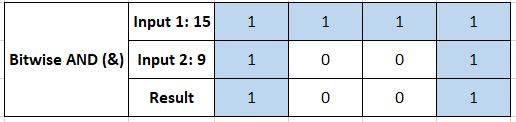• Bitwise OR (|): This operator preforms a binary OR operation; the resultant bit mapping to 1 if one or more of the inputs to it is 1.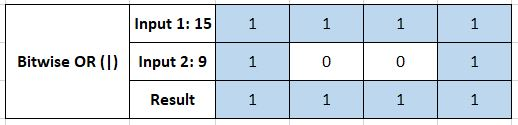• Bitwise XOR (^): Performs the exclusive-OR or XOR operation; the resultant bit maps to 1 if both inputs are not alike (i.e. 0-1 or 1-0) but 0 otherwise.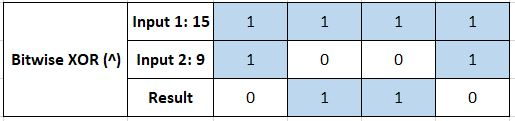• Bitwise Unary NOT (~): Performs complementation or negation operation; inverts all the bits of the number, i.e. 0->1 and 1->0.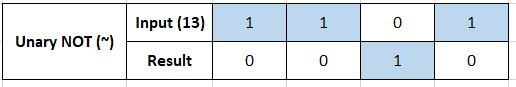• Left Shift (<<): Performs a shift or rotation by a specified number of positions. Every bit is moved left n positions. Further, the MSB (Most Significant Bit) is shifted to the LSB (Least Significant Bit) for every rotation. It is the equivalent of multiplying the number by 2 n times.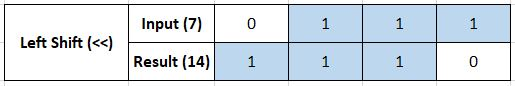• Right Shift (>>): Every bit is moved right n positions. Further, in case of signed 2's complement numbers, the sign bit is moved into the MSB position. It is the equivalent of dividing the number by 2 n times.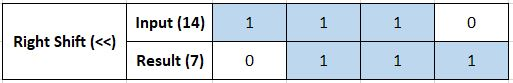### Explanation

Answer is bitwise XOR operation should be zero

Two numbers can be checked for equality even without using the == operator by employing bitwise operators. If you remember, the XOR operation would map to 0s for like bits. If two numbers are the same, they translate to the same bit sequence in binary. This means the application of the bitwise XOR operation should return a resultant sequence of all 0s.

This can be checked programmaticaly through a very simple implementation, as provided here in `Java` and `Python`

• Java
• Python

### Java

``````
public class CheckEqualBitwise
{
public static boolean isEqual(int number1, int number2)
{
return ((number1^number2)==0);
//the xor result is checked
}
public static void main(String []args)
{
System.out.println(isEqual(2,2));
System.out.println(isEqual(2,3));
//tests are conducted for equal and unequal numbers
}
}
``````

### Python

``````
def isEqualBitwise(number1, number2):
return ((number1^number2)==0)
#xor result is checked
print(isEqualBitwise(2,2))
print(isEqualBitwise(2,3))
``````

### Applications

On the surface, it may seem that bit-level operations are trivial tasks incorporated just as an option for the programmer, unlikely to have much real world use. That said, there are some very interesting use-cases for these, as follows:

• They provide scope for set manipulation, where a set is considered as a collection of bits. Then the operations can map to set operations, like | for union, & for intersection, ^ as union-intersection and so on.
• In certain programs and systems, we can use bitwise operators with flags or masks held in a single byte (8 bits for 8 states)
• In computer networks, communication protocols always involve operations like parity checks, checksums, stop bits, flow-control and so on, all of which can work well with logic values (1/0) instead of actual numeric values, considering several networks transmit one bit at a time.
• The XOR operation is a favourite in several encryption and compression schemes.
• Bitwise operations like left or right shift are significantly faster than multiplication or division-like operations. While this may not feel like a significant factor on modern CPUs, it can still be a very important boost in embedded systems or slow processors.
• These operations can be used to manipulate bits in the hardware registers, thus allowing a close interaction with the system.
• They can be used to represent finite state machines
• Bitwise operators found application in old GUI systems as well. The XOR was used to make it easier to redraw certain areas on bitmap screens, for example.#### Ronit Ray

Presently a student on the lookout for any opportunity to widen my horizons. Quick learner, forever willing to adapt and ready to take up any challenge in CS-related fields.

Check if Two Numbers are Equal using Bitwise Operators